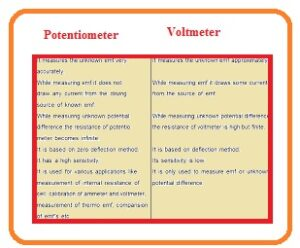Hello, friends, I hope you all are doing great. In today’s tutorial, we will discuss the Difference Between Potentiometer & Voltmeter.  voltmeter and potentiometer both are used to find the value of voltage. a voltmeter is used to find the voltage at the endpoint of circuitry while a potentiometer is to find the electromotive force circuitry. The working operation of the potentiometer is based on the comparison in which the unknown voltage of the circuit at any point can be found by comparing the voltage of the source with that point. If both voltages have a similar value then it shows on the galvanometer.

While the voltmeter is linked in parallel combination to find the value of the voltage of the circuitry. In today’s post, we will have a detailed look at both potentiometer and voltmeter and compare them to find their differences. So let’s get started with the difference between potentiometer and voltmeter.

#### Difference Between Potentiometer & Voltmeter

Potentiometer

• It is resistance having 3 pinouts with rotating points that change the value resistance when employed in circuitry.
• Due to resistance change, it operates as a voltage divider.
• It operates like a rheostat when its two terminals are used in circuitry.
• It is used to measure the value of voltage and works as a voltage divider.
• The tape recorder uses this module to control the voice.
• The sensitivity of this device is larger than the voltmeter.
• It does not have a pointing needle like the voltmeter.
• Its working operation is like the position transducer same operation like to the joystick
• It is not used to regulate the power because it loses more power than another device
• Its structure is simple that consists of a resistance component sliding connection called a wiper.
• Its resistive part is created with carbon, graphite plastic
• Its named pots
• The brightness of tv is regulated through this device
• It provides the exact measured value.
• The resistance value offered by this instrument is infinity.Voltmeter

• The instrument used to find the value of voltage about two terminals of circuitry is called a voltmeter.
• The value of resistance offered by the voltmeter is larger than the ammeter.
• Its resistance value is large than the ammeter.
• Its measuring range can be varied.
• Some meter gets power from an outer source and some have its own power source to operate.
• With a transformer different voltmeters used to show the value of volts at input and output.
• An Analog meter has a needle that indicates the voltage value that to is calculated.
• To measure the small value of voltage it is linked to amplifier circuitry.
• The digital meter indicates voltage in numbers
• The circuit about which voltage is measured voltmeter is connected in parallel combination.
• It is less accurate than the ammeter.
• For measuring the volts value from some volts to thousand volts general-purpose voltmeter used
• The digital meter has a high level of correctness normally one percent than other

### Potentiometer Vs Voltmeter

Potentiometer Voltmeter
Definition It is a device used for the measurement of potential difference by comparing with a known value of voltage it is a device used for the measurement of voltage or electric potential difference
Operation It operates by comparing voltage loss about known resistance with voltage calculated it operated by connecting in circuits with parallel configurations and measuring volts directly
Types its main types are linear, rotary, digital it has Analog and digital meter types
Components its components are resistive elements, wipers, terminals Moving coil or digital display, input terminals
Applications it is used for instruments calibration, measurement of resistance, balancing of bridge circuits It is used for voltage in circuits calculation, testing batteries, power supplies
Sensitivity High High
Range Wide Limited
Accuracy High High
Resolution High High
Units Ohms Volts
Calibration Required Required
Calibration Standard Known resistance Known voltage
Power source None required None required
Interference Minimal Minimal
Cost Low to moderate Low to High
Portable Yes Yes
Safety it has the ability to handle high values of voltage and current it also handles voltage and current
Limitations it measures voltage indirectly It measures voltage directly
Additional features None Its other features are frequency measurement, capacitance measurement, and resistance measurement